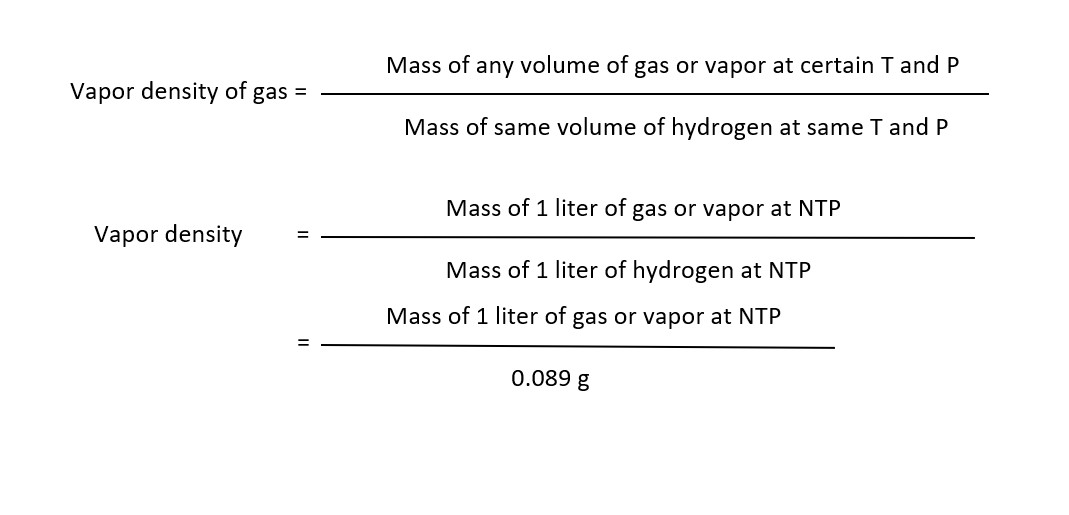# Some Deductions from Avogadro's Law↪ The hypothesis is perfectly general in its application
↪ Avogadro's law is used to deduce atomicity (total number of atoms present in a molecule) of elementary gases.
↪ Keeping this in mind, some of the important relationships are deduced by the application of the law as follows:

﻿To Deduce Molecular Mass = 2 × Vapor DensityThe relative density of a gas or vapor is the ratio of mass of volume of the gas to mass of equal volume of hydrogen under same conditions.According to Avogadro's hypothesis, same volume at the same temperature and pressure contain same number of molecules (n say)Since, Avogadro proved that elementary gases such as hydrogen etc. as diatomic molecules,Molecular mass = 2 x Vapor density or M = 2 x V.D.

⁕ ﻿Molar Volume is 22.4L at NTP

﻿As we know that,﻿Since, 1 liter hydrogen at NTP weighs 0.089 g.
Therefore,

Mass of 1 liter of gas or vapor at NTP = 0.089  × Its vapor density = 0.089  × (M/2)
i.e., 0.089 × (M/2) g of gas vapor occupy a volume of 1 liter at NTP.

So, M g of gas or vapor occupies a volume of (2/0.089) or 22.4 L at NTP.

i.e., one gram-molecular mass of any gas or vapor occupies 22.4 L at NTP.
↪ This is called the gram molecular volume or simply the molar volume.